## GPU Gems 3

The CD content, including demos and content, is available on the web and for download.

You can also subscribe to our Developer News Feed to get notifications of new material on the site.

# Chapter 31. Fast N-Body Simulation with CUDA

Lars Nyland
NVIDIA Corporation

Mark Harris
NVIDIA Corporation

Jan Prins
University of North Carolina at Chapel Hill

## 31.1 Introduction

An N-body simulation numerically approximates the evolution of a system of bodies in which each body continuously interacts with every other body. A familiar example is an astrophysical simulation in which each body represents a galaxy or an individual star, and the bodies attract each other through the gravitational force, as in Figure 31-1. N-body simulation arises in many other computational science problems as well. For example, protein folding is studied using N-body simulation to calculate electrostatic and van der Waals forces. Turbulent fluid flow simulation and global illumination computation in computer graphics are other examples of problems that use N-body simulation.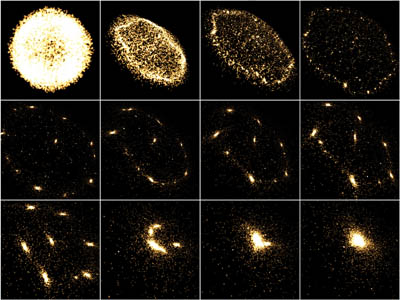Figure 31-1 Frames from an Interactive 3D Rendering of a 16,384-Body System Simulated by Our Application

The all-pairs approach to N-body simulation is a brute-force technique that evaluates all pair-wise interactions among the N bodies. It is a relatively simple method, but one that is not generally used on its own in the simulation of large systems because of its O(N 2) computational complexity. Instead, the all-pairs approach is typically used as a kernel to determine the forces in close-range interactions. The all-pairs method is combined with a faster method based on a far-field approximation of longer-range forces, which is valid only between parts of the system that are well separated. Fast N-body algorithms of this form include the Barnes-Hut method (BH) (Barnes and Hut 1986), the fast multipole method (FMM) (Greengard 1987), and the particle-mesh methods (Hockney and Eastwood 1981, Darden et al. 1993).

The all-pairs component of the algorithms just mentioned requires substantial time to compute and is therefore an interesting target for acceleration. Improving the performance of the all-pairs component will also improve the performance of the far-field component as well, because the balance between far-field and near-field (all-pairs) can be shifted to assign more work to a faster all-pairs component. Accelerating one component will offload work from the other components, so the entire application benefits from accelerating one kernel.

In this chapter, we focus on the all-pairs computational kernel and its implementation using the NVIDIA CUDA programming model. We show how the parallelism available in the all-pairs computational kernel can be expressed in the CUDA model and how various parameters can be chosen to effectively engage the full resources of the NVIDIA GeForce 8800 GTX GPU. We report on the performance of the all-pairs N-body kernel for astrophysical simulations, demonstrating several optimizations that improve performance. For this problem, the GeForce 8800 GTX calculates more than 10 billion interactions per second with N = 16,384, performing 38 integration time steps per second. At 20 flops per interaction, this corresponds to a sustained performance in excess of 200 gigaflops. This result is close to the theoretical peak performance of the GeForce 8800 GTX GPU.

## 31.2 All-Pairs N-Body Simulation

We use the gravitational potential to illustrate the basic form of computation in an all-pairs N-body simulation. In the following computation, we use bold font to signify vectors (typically in 3D). Given N bodies with an initial position x i and velocity v i for 1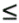iN, the force vector f ij on body i caused by its gravitational attraction to body j is given by the following: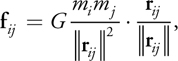where mi and mj are the masses of bodies i and j, respectively; r ij = x j - x i is the vector from body i to body j; and G is the gravitational constant. The left factor, the magnitude of the force, is proportional to the product of the masses and diminishes with the square of the distance between bodies i and j. The right factor is the direction of the force, a unit vector from body i in the direction of body j (because gravitation is an attractive force).

The total force F i on body i, due to its interactions with the other N - 1 bodies, is obtained by summing all interactions: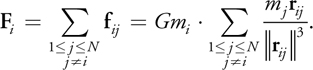As bodies approach each other, the force between them grows without bound, which is an undesirable situation for numerical integration. In astrophysical simulations, collisions between bodies are generally precluded; this is reasonable if the bodies represent galaxies that may pass right through each other. Therefore, a softening factor 2 > 0 is added, and the denominator is rewritten as follows: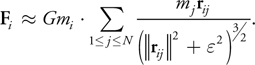Note the condition j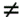i is no longer needed in the sum, because f ii = 0 when 2 > 0. The softening factor models the interaction between two Plummer point masses: masses that behave as if they were spherical galaxies (Aarseth 2003, Dyer and Ip 1993). In effect, the softening factor limits the magnitude of the force between the bodies, which is desirable for numerical integration of the system state.

To integrate over time, we need the acceleration a i = F i /mi to update the position and velocity of body i, and so we simplify the computation to this: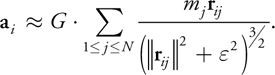The integrator used to update the positions and velocities is a leapfrog-Verlet integrator (Verlet 1967) because it is applicable to this problem and is computationally efficient (it has a high ratio of accuracy to computational cost). The choice of integration method in N-body problems usually depends on the nature of the system being studied. The integrator is included in our timings, but discussion of its implementation is omitted because its complexity is O(N) and its cost becomes insignificant as N grows.

## 31.3 A CUDA Implementation of the All-Pairs N-Body Algorithm

We may think of the all-pairs algorithm as calculating each entry f ij in an NxN grid of all pair-wise forces.  Then the total force F i (or acceleration a i ) on body i is obtained from the sum of all entries in row i. Each entry can be computed independently, so there is O(N 2) available parallelism. However, this approach requires O(N 2) memory and would be substantially limited by memory bandwidth. Instead, we serialize some of the computations to achieve the data reuse needed to reach peak performance of the arithmetic units and to reduce the memory bandwidth required.

Consequently, we introduce the notion of a computational tile, a square region of the grid of pair-wise forces consisting of p rows and p columns. Only 2p body descriptions are required to evaluate all p 2 interactions in the tile (p of which can be reused later). These body descriptions can be stored in shared memory or in registers. The total effect of the interactions in the tile on the p bodies is captured as an update to p acceleration vectors.

To achieve optimal reuse of data, we arrange the computation of a tile so that the interactions in each row are evaluated in sequential order, updating the acceleration vector, while the separate rows are evaluated in parallel. In Figure 31-2, the diagram on the left shows the evaluation strategy, and the diagram on the right shows the inputs and outputs for a tile computation.

In the remainder of this section, we follow a bottom-up presentation of the full computation, packaging the available parallelism and utilizing the appropriate local memory at each level.

#### 31.3.1 Body-Body Force Calculation

The interaction between a pair of bodies as described in Section 31.2 is implemented as an entirely serial computation. The code in Listing 31-1 computes the force on body i from its interaction with body j and updates acceleration a i of body i as a result of this interaction. There are 20 floating-point operations in this code, counting the additions, multiplications, the sqrtf() call, and the division (or reciprocal).

#### Example 31-1. Updating Acceleration of One Body as a Result of Its Interaction with Another Body

`    __device__ float3 bodyBodyInteraction(float4 bi, float4 bj, float3 ai) {   float3 r;   // r_ij  [3 FLOPS]   r.x = bj.x - bi.x;   r.y = bj.y - bi.y;   r.z = bj.z - bi.z;   // distSqr = dot(r_ij, r_ij) + EPS^2  [6 FLOPS]    float distSqr = r.x * r.x + r.y * r.y + r.z * r.z + EPS2;   // invDistCube =1/distSqr^(3/2)  [4 FLOPS (2 mul, 1 sqrt, 1 inv)]    float distSixth = distSqr * distSqr * distSqr;   float invDistCube = 1.0f/sqrtf(distSixth);   // s = m_j * invDistCube [1 FLOP]    float s = bj.w * invDistCube;   // a_i =  a_i + s * r_ij [6 FLOPS]   ai.x += r.x * s;   ai.y += r.y * s;   ai.z += r.z * s;   return ai; } `

We use CUDA's float4 data type for body descriptions and accelerations stored in GPU device memory. We store each body's mass in the w field of the body's float4 position. Using float4 (instead of float3) data allows coalesced memory access to the arrays of data in device memory, resulting in efficient memory requests and transfers. (See the CUDA Programming Guide (NVIDIA 2007) for details on coalescing memory requests.) Three-dimensional vectors stored in local variables are stored as float3 variables, because register space is an issue and coalesced access is not.

#### 31.3.2 Tile Calculation

A tile is evaluated by p threads performing the same sequence of operations on different data. Each thread updates the acceleration of one body as a result of its interaction with p other bodies. We load p body descriptions from the GPU device memory into the shared memory provided to each thread block in the CUDA model. Each thread in the block evaluates p successive interactions. The result of the tile calculation is p updated accelerations.

The code for the tile calculation is shown in Listing 31-2. The input parameter myPosition holds the position of the body for the executing thread, and the array shPosition is an array of body descriptions in shared memory. Recall that p threads execute the function body in parallel, and each thread iterates over the same p bodies, computing the acceleration of its individual body as a result of interaction with p other bodies.

#### Example 31-2. Evaluating Interactions in a pxp Tile

`    __device__ float3 tile_calculation(float4 myPosition, float3 accel) {   int i;   extern __shared__ float4[] shPosition;   for (i = 0; i < blockDim.x; i++) {     accel = bodyBodyInteraction(myPosition, shPosition[i], accel);   }   return accel; } `

The G80 GPU architecture supports concurrent reads from multiple threads to a single shared memory address, so there are no shared-memory-bank conflicts in the evaluation of interactions. (Refer to the CUDA Programming Guide (NVIDIA 2007) for details on the shared memory broadcast mechanism used here.)

#### 31.3.3 Clustering Tiles into Thread Blocks

We define a thread block as having p threads that execute some number of tiles in sequence. Tiles are sized to balance parallelism with data reuse. The degree of parallelism (that is, the number of rows) must be sufficiently large so that multiple warps can be interleaved to hide latencies in the evaluation of interactions. The amount of data reuse grows with the number of columns, and this parameter also governs the size of the transfer of bodies from device memory into shared memory. Finally, the size of the tile also determines the register space and shared memory required. For this implementation, we have used square tiles of size p by p. Before executing a tile, each thread fetches one body into shared memory, after which the threads synchronize. Consequently, each tile starts with p successive bodies in the shared memory.

Figure 31-3 shows a thread block that is executing code for multiple tiles. Time spans the horizontal direction, while parallelism spans the vertical direction. The heavy lines demarcate the tiles of computation, showing where shared memory is loaded and a barrier synchronization is performed. In a thread block, there are N/p tiles, with p threads computing the forces on p bodies (one thread per body). Each thread computes all N interactions for one body.

The code to calculate N-body forces for a thread block is shown in Listing 31-3. This code is the CUDA kernel that is called from the host.

The parameters to the function calculate_forces() are pointers to global device memory for the positions devX and the accelerations devA of the bodies. We assign them to local pointers with type conversion so they can be indexed as arrays. The loop over the tiles requires two synchronization points. The first synchronization ensures that all shared memory locations are populated before the gravitation computation proceeds, and the second ensures that all threads finish their gravitation computation before advancing to the next tile. Without the second synchronization, threads that finish their part in the tile calculation might overwrite the shared memory still being read by other threads.

#### Example 31-3. The CUDA Kernel Executed by a Thread Block with p Threads to Compute the Gravitational Acceleration for p Bodies as a Result of All N Interactions

`    __global__ void calculate_forces(void *devX, void *devA) {   extern __shared__ float4[] shPosition;   float4 *globalX = (float4 *)devX;   float4 *globalA = (float4 *)devA;   float4 myPosition;   int i, tile;   float3 acc = {0.0f, 0.0f, 0.0f};   int gtid = blockIdx.x * blockDim.x + threadIdx.x;   myPosition = globalX[gtid];   for (i = 0, tile = 0; i < N; i += p, tile++) {     int idx = tile * blockDim.x + threadIdx.x;     shPosition[threadIdx.x] = globalX[idx];     __syncthreads();     acc = tile_calculation(myPosition, acc);     __syncthreads();   }   // Save the result in global memory for the integration step.    float4 acc4 = {acc.x, acc.y, acc.z, 0.0f};   globalA[gtid] = acc4; } `

#### 31.3.4 Defining a Grid of Thread Blocks

The kernel program in Listing 31-3 calculates the acceleration of p bodies in a system, caused by their interaction with all N bodies in the system. We invoke this program on a grid of thread blocks to compute the acceleration of all N bodies. Because there are p threads per block and one thread per body, the number of thread blocks needed to complete all N bodies is N/p, so we define a 1D grid of size N/p. The result is a total of N threads that perform N force calculations each, for a total of N 2 interactions.

Evaluation of the full grid of interactions can be visualized as shown in Figure 31-4. The vertical dimension shows the parallelism of the 1D grid of N/p independent thread blocks with p threads each. The horizontal dimension shows the sequential processing of N force calculations in each thread. A thread block reloads its shared memory every p steps to share p positions of data.

## 31.4 Performance Results

By simply looking at the clocks and capacities of the GeForce 8800 GTX GPU, we observe that it is capable of 172.8 gigaflops (128 processors, 1.35 GHz each, one floatingpoint operation completed per cycle per processor). Multiply-add instructions (MADs) perform two floating-point operations every clock cycle, doubling the potential performance. Fortunately, the N-body code has several instances where MAD instructions are generated by the compiler, raising the performance ceiling well over 172.8 gigaflops.

Conversely, complex instructions such as inverse square root require multiple clock cycles. The CUDA Programming Guide (NVIDIA 2007) says to expect 16 clock cycles per warp of 32 threads, or four times the amount of time required for the simpler operations. Our code uses one inverse-square-root instruction per interaction.

When comparing gigaflop rates, we simply count the floating-point operations listed in the high-level code. By counting the floating-point operations in the bodyBodyInteraction code (Listing 31-1), we see nine additions, nine multiplications, one square root, and one division. Division and square root clearly require more time than addition or multiplication, and yet we still assign a cost of 1 flop each,  yielding a total of 20 floating-point operations per pair-wise force calculation. This value is used throughout the chapter to compute gigaflops from interactions per second.

#### 31.4.1 Optimization

Our first implementation achieved 163 gigaflops for 16,384 bodies. This is an excellent result, but there are some optimizations we can use that will increase the performance.

#### Performance Increase with Loop Unrolling

The first improvement comes from loop unrolling, where we replace a single body-body interaction call in the inner loop with 2 to 32 calls to reduce loop overhead. A chart of performance for small unrolling factors is shown in Figure 31-5.

We examined the code generated by the CUDA compiler for code unrolled with 4 successive calls to the body-body interaction function. It contains 60 instructions for the 4 in-lined calls. Of the 60 instructions, 56 are floating-point instructions, containing 20 multiply-add instructions and 4 inverse-square-root instructions. Our best hope is that the loop will require 52 cycles for the non-inverse-square-root floating-point instructions, 16 cycles for the 4 inverse-square-root instructions, and 4 cycles for the loop control, totaling 72 cycles to perform 80 floating-point operations.

If this performance is achieved, the G80 GPU will perform approximately 10 billion body-body interactions per second (128 processors at 1350 MHz, computing 4 bodybody interactions in 72 clock cycles), or more than 200 gigaflops. This is indeed the performance we observe for N > 8192, as shown in Figure 31-6.

#### Performance Increase as Block Size Varies

Another performance-tuning parameter is the value of p, the size of the tile. The total memory fetched by the program is N 2/p for each integration time step of the algorithm, so increasing p decreases memory traffic. There are 16 multiprocessors on the GeForce 8800 GTX GPU, so p cannot be arbitrarily large; it must remain small enough so that N/p is 16 or larger. Otherwise, some multiprocessors will be idle.

Another reason to keep p small is the concurrent assignment of thread blocks to multiprocessors. When a thread block uses only a portion of the resources on a multiprocessor (such as registers, thread slots, and shared memory), multiple thread blocks are placed on each multiprocessor. This technique provides more opportunity for the hardware to hide latencies of pipelined instruction execution and memory fetches. Figure 31-7 shows how the performance changes as p is varied for N = 1024, 4096, and 16,384.

#### Improving Performance for Small N

A final optimization that we implemented—using multiple threads per body—attempts to improve performance for N < 4096. As N decreases, there is not enough work with one thread per body to adequately cover all the latencies in the GeForce 8800 GTX GPU, so performance drops rapidly. We therefore increase the number of active threads by using multiple threads on each row of a body's force calculation. If the additional threads are part of the same thread block, then the number of memory requests increases, as does the number of warps, so the latencies begin to be covered again. Our current register use limits the number of threads per block to 256 on the 8800 GTX GPU (blocks of 512 threads fail to run), so we split each row into q segments, keeping p x q256.

Splitting the rows has the expected benefit. Using two threads to divide the work increased performance for N = 1024 by 44 percent. The improvement rapidly diminishes when splitting the work further. And of course, for N > 4096, splitting had almost no effect, because the code is running at nearly peak performance. Fortunately, splitting did not reduce performance for large N. Figure 31-8 shows a graph demonstrating the performance gains.

#### 31.4.2 Analysis of Performance Results

When we judge the performance gain of moving to the GeForce 8800 GTX GPU, the most surprising and satisfying result is the speedup of the N-body algorithm compared to its performance on a CPU. The performance is much larger than the comparison of peak floating-point rates between the GeForce 8800 GTX GPU and Intel processors. We speculate that the main reason for this gain is that Intel processors require dozens of unpipelined clock cycles for the division and square root operations, whereas the GPU has a single instruction that performs an inverse square root. Intel's Streaming SIMD Extensions (SSE) instruction set includes a four-clock-cycle 1/sqrt(x) instruction (vector and scalar), but the accuracy is limited to 12 bits. In a technical report from Intel (Intel 1999), a method is proposed to increase the accuracy over a limited domain, but the cost is estimated to be 16 clock cycles.

## 31.5 Previous Methods Using GPUs for N-Body Simulation

The N-body problem has been studied throughout the history of computing. In the 1980s several hierarchical and mesh-style algorithms were introduced, successfully reducing the O(N 2) complexity. The parallelism of the N-body problem has also been studied as long as there have been parallel computers. We limit our review to previous work that pertains to achieving high performance using GPU hardware.

In 2004 we built an N-body application for GPUs by using Cg and OpenGL (Nyland, Harris, and Prins 2004). Although Cg presented a more powerful GPU programming language than had previously been available, we faced several drawbacks to building the application in a graphics environment. All data were either read-only or write-only, so a double-buffering scheme had to be used. All computations were initiated by drawing a rectangle whose pixel values were computed by a shader program, requiring O(N 2) memory. Because of the difficulty of programming complex algorithms in the graphics API, we performed simple brute-force computation of all pair-wise accelerations into a single large texture, followed by a parallel sum reduction to get the vector of total accelerations. This sum reduction was completely bandwidth bound because of the lack of on-chip shared memory. The maximum texture-size limitation of the GPU limited the largest number of bodies we could handle (at once) to 2048. Using an out-of-core method allowed us to surpass that limit.

A group at Stanford University (Elsen et al. 2006) created an N-body solution similar to the one described in this chapter, using the BrookGPU programming language (Buck et al. 2004), gathering performance data from execution on an ATI X1900 XTX GPU. They concluded that loop unrolling significantly improves performance. They also concluded that achieving good performance when N < 4096 is difficult and suggest a similar solution to ours, achieving similar improvement. The Stanford University group compares their GPU implementation to a highly tuned CPU implementation (SSE assembly language that achieves 3.8 gigaflops, a performance metric we cannot match) and observe the GPU outperforming the CPU by a factor of 25. They provide code (written in BrookGPU) and analyze what the code and the hardware are doing. The GPU hardware they used achieves nearly 100 gigaflops. They also remind us that the CPU does half the number of force calculations of the GPU by using the symmetry of f ij = -f ji .

Since the release of the GeForce 8800 GTX GPU and CUDA, several implementations of N-body applications have appeared. Two that caught our attention are Hamada and Iitaka 2007 and Portegies Zwart et al. 2007. Both implementations mimic the Gravity Pipe (GRAPE) hardware (Makino et al. 2000), suggesting that the GeForce 8800 GTX GPU replace the GRAPE custom hardware. Their N-body method uses a multiple time-step scheme, with integration steps occurring at different times for different bodies, so the comparison with these two methods can only be done by comparing the number of pair-wise force interactions per second. We believe that the performance we have achieved is nearly two times greater than the performance of the cited works.

## 31.6 Hierarchical N-Body Methods

Many practical N-body applications use a hierarchical approach, recursively dividing the space into subregions until some criterion is met (for example, that the space contains fewer than k bodies). For interactions within a leaf cell, the all-pairs method is used, usually along with one or more layers of neighboring leaf cells. For interactions with subspaces farther away, far-field approximations are used. Popular hierarchical methods are Barnes-Hut (Barnes and Hut 1986) and Greengard's fast multipole method (Greengard 1987, Greengard and Huang 2002).

Both algorithms must choose how to interact with remote leaf cells. The general result is that many body-cell or cell-cell interactions require an all-pairs solution to calculate the forces. The savings in the algorithm comes from the use of a multipole expansion of the potential due to bodies at a distance, rather than from interactions with the individual bodies at a distance.

As an example in 3D, consider a simulation of 218 bodies (256 K), decomposed into a depth-3 octree containing 512 leaf cells with 512 bodies each. The minimum neighborhood of cells one layer deep will contain 27 leaf cells, but probably many more will be used. For each leaf cell, there are at least 27 x 512 x 512 pair-wise force interactions to compute. That yields more than 7 million interactions per leaf cell, which in our implementation would require less than 1 millisecond of computation to solve. The total time required for all 512 leaf cells would be less than a half-second.

Contrast this with our all-pairs implementation  on an Intel Core 2 Duo  that achieves about 20 million interactions per second. The estimated time for the same calculation is about 90 seconds (don't forget that the CPU calculates only half as many pair-wise interactions). Even the high-performance implementations that compute 100 million interactions per second require 18 seconds. One way to alleviate the load is to deepen the hierarchical decomposition and rely more on the far-field approximations, so that the leaf cells would be populated with fewer particles. Of course, the deeper tree means more work in the far-field segment.

We believe that the savings of moving from the CPU to the GPU will come not only from the increased computational horsepower, but also from the increased size of the leaf cells, making the hierarchical decomposition shallower, saving time in the far-field evaluation as well. In future work we hope to implement the BH or FMM algorithms, to evaluate the savings of more-efficient algorithms.

## 31.7 Conclusion

It is difficult to imagine a real-world algorithm that is better suited to execution on the G80 architecture than the all-pairs N-body algorithm. In this chapter we have demonstrated three features of the algorithm that help it achieve such high efficiency:

• Straightforward parallelism with sequential memory access patterns
• Data reuse that keeps the arithmetic units busy
• Fully pipelined arithmetic, including complex operations such as inverse square root, that are much faster clock-for-clock on a GeForce 8800 GTX GPU than on a CPU

The result is an algorithm that runs more than 50 times as fast as a highly tuned serial implementation (Elsen et al. 2006) or 250 times faster than our portable C implementation. At this performance level, 3D simulations with large numbers of particles can be run interactively, providing 3D visualizations of gravitational, electrostatic, or other mutual-force systems.

## 31.8 References

Aarseth, S. 2003. Gravitational N-Body Simulations. Cambridge University Press.

Barnes, J., and P. Hut. 1986. "A Hierarchical O(n log n) Force Calculation Algorithm." Nature 324.

Buck, I., T. Foley, D. Horn, J. Sugerman, K. Fatahalian, M. Houston, and P. Hanrahan. 2004. "Brook for GPUs: Stream Computing on Graphics Hardware." In ACM Transactions on Graphics (Proceedings of SIGGRAPH 2004) 23(3).

Darden, T., D. York, and L. Pederson. 1993. "Particle Mesh Ewald: An N log(N) Method for Ewald Sums in Large Systems." Journal of Chemical Physics 98(12), p. 10089.

Dehnen, Walter. 2001. "Towards Optimal Softening in 3D N-body Codes: I. Minimizing the Force Error." Monthly Notices of the Royal Astronomical Society 324, p. 273.

Dyer, Charles, and Peter Ip. 1993. "Softening in N-Body Simulations of Collisionless Systems." The Astrophysical Journal 409, pp. 60–67.

Elsen, Erich, Mike Houston, V. Vishal, Eric Darve, Pat Hanrahan, and Vijay Pande. 2006. "N-Body Simulation on GPUs." Poster presentation. Supercomputing 06 Conference.

Greengard, L. 1987. The Rapid Evaluation of Potential Fields in Particle Systems. ACM Press.

Greengard, Leslie F., and Jingfang Huang. 2002. "A New Version of the Fast Multipole Method for Screened Coulomb Interactions in Three Dimensions." Journal of Computational Physics 180(2), pp. 642–658.

Hamada, T., and T. Iitaka. 2007. "The Chamomile Scheme: An Optimized Algorithm for N-body Simulations on Programmable Graphics Processing Units." ArXiv Astrophysics e-prints, astro-ph/0703100, March 2007.

Hockney, R., and J. Eastwood. 1981. Computer Simulation Using Particles. McGraw-Hill.

Intel Corporation. 1999. "Increasing the Accuracy of the Results from the Reciprocal and Reciprocal Square Root Instructions Using the Newton-Raphson Method." Version 2.1. Order Number: 243637-002. Available online at http://cache-www.intel.com/cd/00/00/04/10/41007_nrmethod.pdf.

Intel Corporation. 2003. Intel Pentium 4 and Intel Xeon Processor Optimization Reference Manual. Order Number: 248966-007.

Johnson, Vicki, and Alper Ates. 2005. "NBodyLab Simulation Experiments with GRAPE-6a and MD-GRAPE2 Acceleration." Astronomical Data Analysis Software and Systems XIV P3-1-6, ASP Conference Series, Vol. XXX, P. L. Shopbell, M. C. Britton, and R. Ebert, eds. Available online at http://nbodylab.interconnect.com/docs/P3.1.6_revised.pdf.

Makino, J., T. Fukushige, and M. Koga. 2000. "A 1.349 Tflops Simulation of Black Holes in a Galactic Center on GRAPE-6." In Proceedings of the 2000 ACM/IEEE Conference on Supercomputing.

NVIDIA Corporation. 2007. NVIDIA CUDA Compute Unified Device Architecture Programming Guide. Version 0.8.1.

Nyland, Lars, Mark Harris, and Jan Prins. 2004. "The Rapid Evaluation of Potential Fields Using Programmable Graphics Hardware." Poster presentation at GP2, the ACM Workshop on General Purpose Computing on Graphics Hardware.

Portegies Zwart, S., R. Belleman, and P. Geldof. 2007. "High Performance Direct Gravitational N-body Simulations on Graphics Processing Unit." ArXiv Astrophysics e-prints, astro-ph/0702058, Feb. 2007.

Verlet, J. 1967. "Computer Experiments on Classical Fluids." Physical Review 159(1), pp. 98–103.